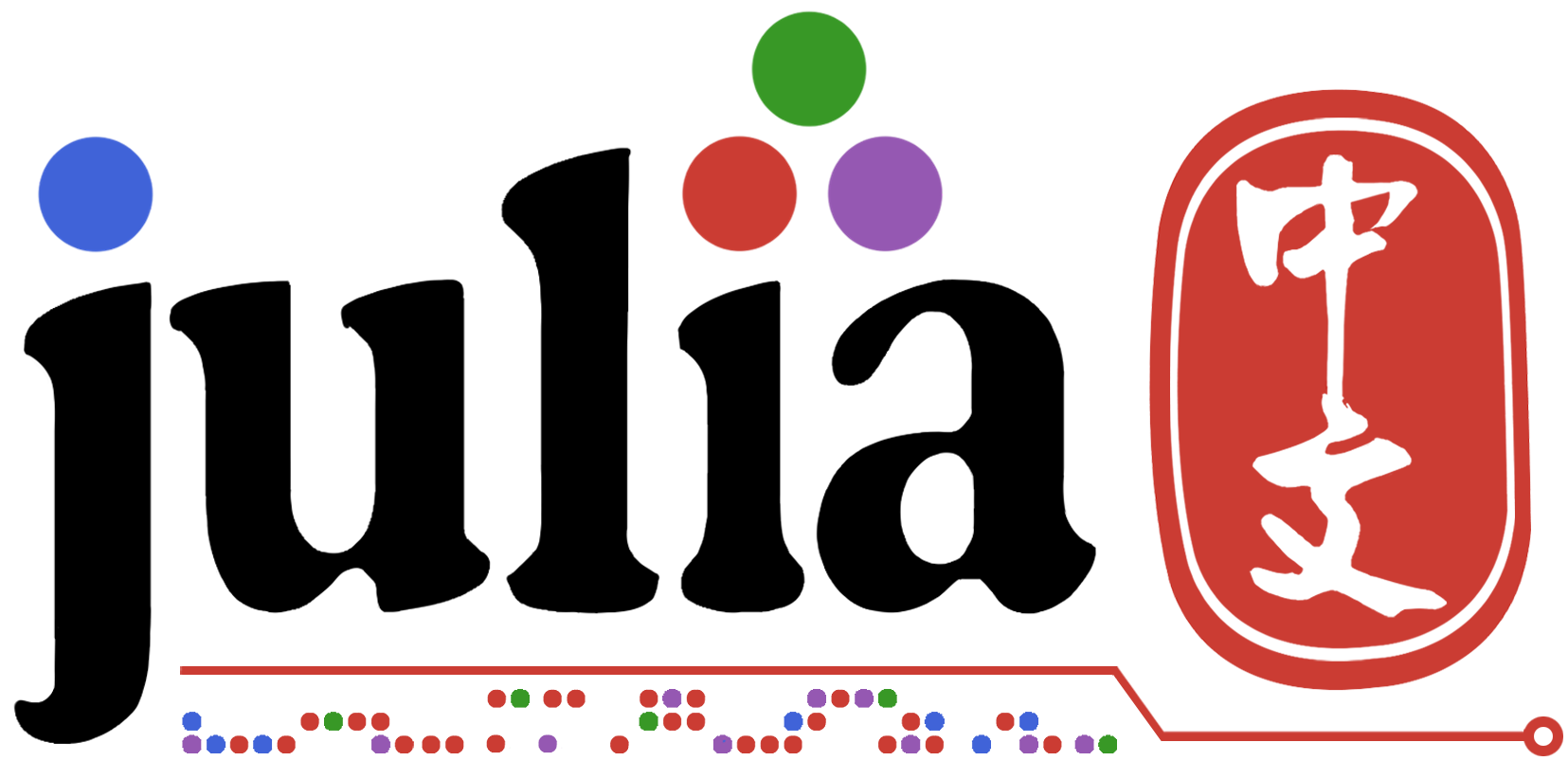# https://github.com/JuliaCN/LeetCode.jl

2赞

+1 id: `@inkydragon`

1赞

``````# 1207. 独一无二的出现次数
# 给你一个整数数组 arr，请你帮忙统计数组中每个数的出现次数。
# 如果每个数的出现次数都是独一无二的，就返回 true；否则返回 false。

function uniqueOccurrences(nums::Vector{Int})::Bool
records = Dict{Int, Int}()
combinefn1(r, x) = begin
r[x] = get(r, x, 0) + 1
r
end

reduce(combinefn1, nums; init = records)

times = Set{Int}()
combinefn2(r, x) = push!(r, x)
reduce(combinefn2, values(records); init = times)

return length(times) == length(records)
end
````````````import Pkg